# Localize and Read Multiple Barcodes in Image

This example shows how to use the `readBarcode` function from the Computer Vision Toolbox™ to detect and decode 1-D and 2-D barcodes in an image. Barcodes are widely used to encode data in a visual, machine-readable format. They are useful in many applications such as item identification, warehouse inventory tracking, and compliance tracking. For 1-D barcodes, the `readBarcode` function returns the location of the barcode endpoints. For 2-D barcodes, the function returns the locations of the finder patterns. This example uses two approaches for localizing multiple barcodes in an image. One approach is clustering-based, which is more robust to different imaging conditions and requires the Statistics and Machine Learning Toolbox™. The second approach uses a segmentation-based workflow and might require parameter tuning based on the imaging conditions.

### Barcode Detection using the `readBarcode` Function

Read a QR code from an image.

```I = imread("barcodeQR.jpg"); % Search the image for a QR Code. [msg, ~, loc] = readBarcode(I); % Annotate the image with the decoded message. xyText = loc(2,:); Imsg = insertText(I, xyText, msg, "BoxOpacity", 1, "FontSize", 25); % Insert filled circles at the finder pattern locations. Imsg = insertShape(Imsg, "filled-circle", [loc, ... repmat(10, length(loc), 1)], "Color", "red", "Opacity", 1); % Display image. imshow(Imsg)```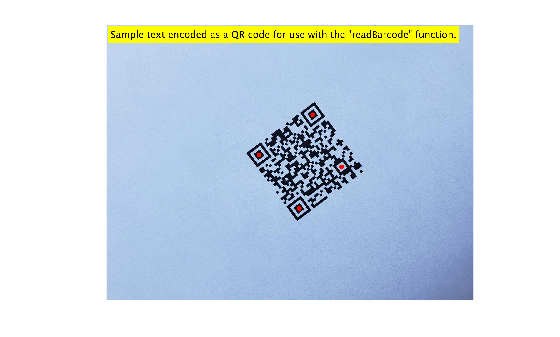Read a 1-D barcode from an image.

```I = imread("barcode1D.jpg"); % Read the 1-D barcode and determine the format.. [msg, format, locs] = readBarcode(I); % Display the detected message and format. disp("Detected format and message: " + format + ", " + msg)```
```Detected format and message: EAN-13, 1234567890128 ```
```% Insert a line to show the scan row of the barcode. xyBegin = locs(1,:); imSize = size(I); I = insertShape(I,"line",[1 xyBegin(2) imSize(2) xyBegin(2)], ... "LineWidth", 7); % Insert markers at the end locations of the barcode. I = insertShape(I, "filled-circle", [locs, ... repmat(10, length(locs), 1)], "Color", "red", "Opacity", 1); % Display image. imshow(I)```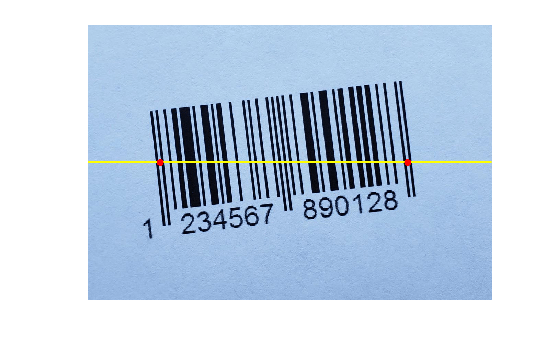### Improving Barcode Detection

For a successful detection, the barcode must be clearly visible. The barcode must also be as closely aligned to a horizontal or vertical position as possible. The `readBarcode` function is inherently more robust to rotations for 2-D or matrix codes than it is to 1-D or linear barcodes. For example, the barcode cannot be detected in this image.

```I = imread("rotated1DBarcode.jpg"); % Display the image. imshow(I)```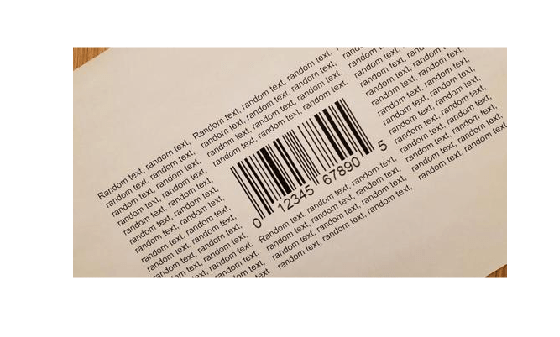```% Pass the image to the readBarcode function. readBarcode(I)```
```ans = "" ```

Rotate the image using the `imrotate` so that the barcode is roughly horizontal. Use `readBarcode` on the rotated image.

```% Rotate the image by 30 degrees clockwise. Irot = imrotate(I, -30); % Display the rotated image. imshow(Irot)```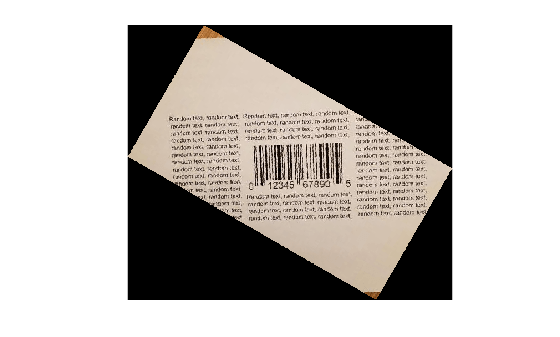```% Pass the rotated image to the readBarcode function. readBarcode(Irot)```
```ans = "012345678905" ```

### Detect Multiple Barcodes

The `readBarcode` function detects only a single barcode in each image. In order to detect multiple barcodes, you must specify a region-of-interest (ROI). To specify an ROI, you can use the `drawrectangle` function to interactively determine the ROIs. You can also use image analysis techniques to detect the ROI of multiple barcodes in the image.

#### Interactively determine ROIs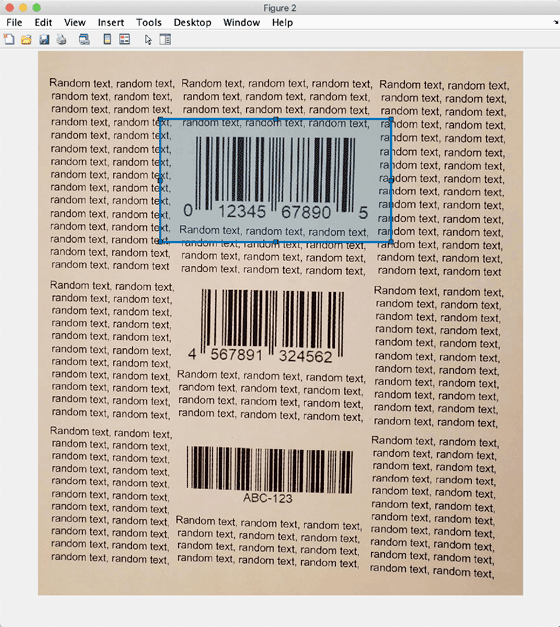`I = imread("multiple1DBarcodes.jpg");`

Use the `drawrectangle` function to draw and obtain rectangle parameters.

` roi1 = drawrectangle;`

` pos = roi1.Position;`

```% ROIs obtained using drawrectangle roi = [180 100 330 180 180 320 330 180 180 550 330 180]; imSize = size(I); for i = 1:size(roi,1) [msg, format, locs] = readBarcode(I, roi(i,:)); disp("Decoded format and message: " + format + ", " + msg) % Insert a line to indicate the scan row of the barcode. xyBegin = locs(1,:); I = insertShape(I,"line",[1 xyBegin(2) imSize(2) xyBegin(2)], ... "LineWidth", 5); % Annotate image with decoded message. I = insertText(I, xyBegin, msg, "BoxOpacity", 1, "FontSize", 20); end```
```Decoded format and message: UPC-A, 012345678905 Decoded format and message: EAN-13, 4567891324562 Decoded format and message: CODE-39, ABC-123 ```
`imshow(I)`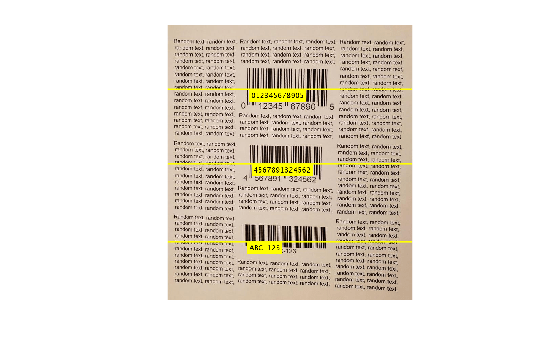#### Image analysis to determine ROIs

Use image analysis techniques to automate the detection of multiple barcodes. This requires localizing multiple barcodes in an image, determining their orientation, and correcting for the orientation. Without preprocessing, barcodes cannot be detected in the image containing multiple rotated barcodes.

```I = imread("multiple1DBarcodesRotated.jpg"); Igray = im2gray(I); % Display the image. imshow(I)```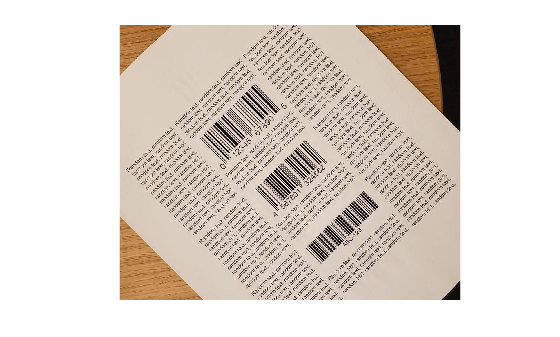```% Pass the unprocessed image to the readBarcode function. readBarcode(Igray, '1D')```
```ans = "" ```

Detection on the unprocessed image resulted in no detection.

Step 1: Detect candidate regions for the barcodes using MSER

Detect regions of interest in the image using the `detectMSERFeatures` function. Then, you can eliminate regions of interest based on a specific criteria such as the aspect ratio. You can use the binary image from the filtered results for further processing.

```% Detect MSER features. [~, cc] = detectMSERFeatures(Igray); % Compute region properties MajorAxisLength and MinorAxisLength. regionStatistics = regionprops(cc, 'MajorAxisLength', 'MinorAxisLength'); % Filter out components that have a low aspect ratio as unsuitable % candidates for the bars in the barcode. minAspectRatio = 10; candidateRegions = find(([regionStatistics.MajorAxisLength]./[regionStatistics.MinorAxisLength]) > minAspectRatio); % Binary image to store the filtered components. BW = false(size(Igray)); % Update the binary image. for i = 1:length(candidateRegions) BW(cc.PixelIdxList{candidateRegions(i)}) = true; end % Display the binary image with the filtered components. imshow(BW) title("Candidate regions for the barcodes")```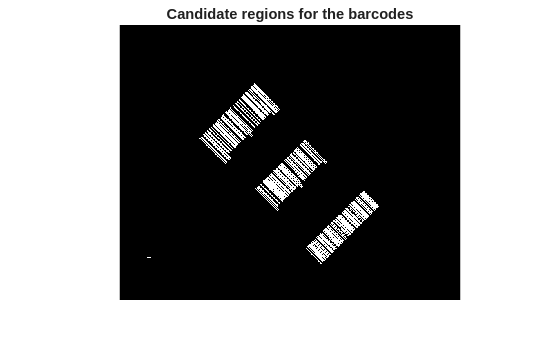Step 2: Extract barcode line segments using hough transform

Detect prominent edges in the image using the `edge` function. Then use the hough transform to find lines of interest. The lines represent possible candidates for the vertical bars in the barcode.

```% Perform hough transform. BW = edge(BW,'canny'); [H,T,R] = hough(BW); % Display the result of the edge detection operation. imshow(BW)```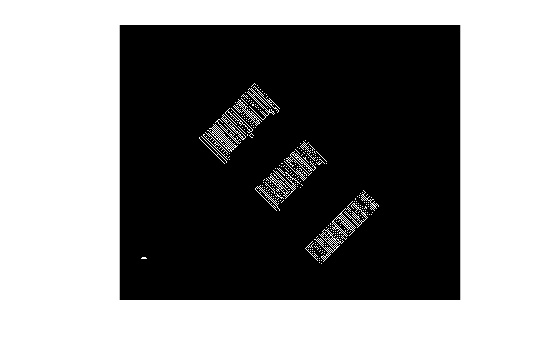```% Determine the size of the suppression neighborhood. reductionRatio = 500; nhSize = floor(size(H)/reductionRatio); idx = mod(nhSize,2) < 1; nhSize(idx) = nhSize(idx) + 1; % Identify the peaks in the Hough transform. P = houghpeaks(H,length(candidateRegions),'NHoodSize',nhSize); % Detect the lines based on the detected peaks. lines = houghlines(BW,T,R,P); % Display the lines detected using the houghlines function. Ihoughlines = ones(size(BW)); % Start and end points of the detected lines. startPts = reshape([lines(:).point1], 2, length(lines))'; endPts = reshape([lines(:).point2], 2, length(lines))'; Ihoughlines = insertShape(Ihoughlines, 'line', [startPts, endPts], ... 'LineWidth', 2, 'Color', 'green'); % Display the original image overlayed with the detected lines. Ibarlines = imoverlay(I, ~Ihoughlines(:,:,1)); imshow(Ibarlines)```Step 3: Localize barcodes in image

After extracting the line segments, two methods are presented for localizing the individual barcodes in the image:

• Method 1: A clustering-based technique that uses functionalities from the Statistics and Machine Learning Toolbox™ to identify individual barcodes. This technique is more robust to outliers that were detected using the image analysis techniques above. It can also be extended to a wide range of imaging conditions without having to tune parameters.

• Method 2: A segmentation-based workflow to separate the individual barcodes. This method uses other image analysis techniques to localize and rotation correct the extracted barcodes. While this works fairly well, it might require some parameter tuning to prevent detection of outliers.

Method 1: Clustering based workflow

There are two steps in this workflow:

1. Determine bisectors of barcode line segments

While it is common practice to directly use the lines (that were obtained using the Hough transform) to localize the barcode, this method uses the lines to further detect the perpendicular bisectors for each of the lines. The bisector lines are represented as points in cartesian space, which makes them suitable for identifying individual barcodes. Using the bisectors make the detection of the individual barcodes more robust, since it results in less misclassifications of lines that are similar but belonging to different barcodes.

2. Perform clustering on the bisectors to identity the individual barcodes

Since all of the bars in a barcode are approximately parallel to each other, the bisectors of each of these bars should ideally be the same line, and their corresponding points should therefore cluster around a single point. In practice, these bisectors will vary from segment to segment, but still remain similar enough to allow the use of a density-based clustering algorithm. The result of performing this clustering operation is a set of clusters, each of which points to a separate barcode. This example uses the `dbscan` (Statistics and Machine Learning Toolbox) function, which does not require prior knowledge of the number of clusters. The different clusters (barcodes) are visualized in this example.

The example checks for a Statistics and Machine Learning Toolbox™ license. If a license is found, the example uses the clustering method. Otherwise, the example uses the segmentation method.

```useClustering = license('test','statistics_toolbox'); if useClustering [boundingBox, orientation, Iclusters] = clusteringLocalization(lines, size(I)); % Display the detected clusters. imshow(Iclusters) else disp("The clustering based workflow requires a license for the Statistics and Machine Learning Toolbox") end```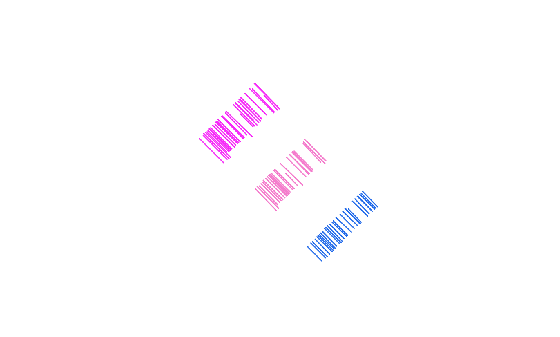Method 2: Segmentation based workflow

Having removed the background noise and variation, the detected vertical bars are grouped into individual barcodes using morphological operations, like `imdilate`. The example uses the `regionprops` function to determine the bounding box and orientation for each of the barcodes. The results are used to crop the individual barcodes from the original image and to orient them to be roughly horizontal.

```if ~useClustering [boundingBox, orientation, Idilated] = segmentationLocalization(Ihoughlines); % Display the dilated image. imshow(Idilated) end```

Step 4: Crop the Barcodes and correct their rotation

The barcodes are cropped from the original image using the bounding boxes obtained from the segmentation. The orientation results are used to align the barcodes to be approximately horizontal.

```% Localize and rotate the barcodes in the image. correctedImages = cell(1, length(orientation)); % Store the cropped and rotation corrected images of the barcodes. for i = 1:length(orientation) I = insertShape(I, 'rectangle', boundingBox(i,:), 'LineWidth',3, 'Color', 'red'); if orientation(i) > 0 orientation(i) = -(90 - orientation(i)); else orientation(i) = 90 + orientation(i); end % Crop the barcode from the original image and rotate it using the % detected orientation. correctedImages{i} = imrotate(imcrop(Igray,boundingBox(i,:)), orientation(i)); end % Display the image with the localized barcodes. imshow(I)```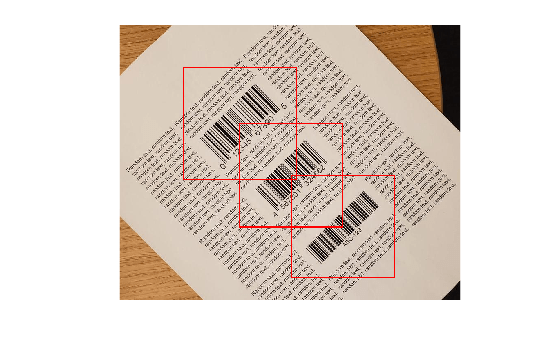Step 5: Detect barcodes in the cropped and rotation corrected images

The cropped and rotation corrected images of the barcodes are then used with the `readBarcode` function to decode them.

```% Pass each of the images to the readBarcode function. for i = 1:length(correctedImages) [msg, format, ~] = readBarcode(correctedImages{i}, '1D'); disp("Decoded format and message: " + format + ", " + msg) end```
```Decoded format and message: UPC-A, 012345678905 Decoded format and message: EAN-13, 4567891324562 Decoded format and message: CODE-39, ABC-123 ```

This example showed how the `readBarcode` function can be used to detect, decode and localize barcodes in an image. While the function works well when the alignment of the barcodes is roughly horizontal or vertical, it needs additional pre-processing when the barcodes appear rotated. The preprocessing steps detailed above is a good starting point to work with multiple barcodes that are not aligned in an image.

#### Supporting Functions

clusteringLocalization uses a clustering-based workflow to localize individual barcodes.

```function [boundingBox, orientation, Iclusters] = clusteringLocalization(lines, imSize) %------------------------------------------------------------------------ % Determine Bisectors of Barcode Line Segments %------------------------------------------------------------------------ % Table to store the properties of the bisectors of the detected lines. linesBisector = array2table(zeros(length(lines), 4), 'VariableNames', {'theta', 'rho', 'x', 'y'}); % Use the orientation values of the lines to determine the orientation. % values of the bisectors idxNeg = find([lines.theta] < 0); idxPos = find([lines.theta] >= 0); negAngles = 90 + [lines(idxNeg).theta]; linesBisector.theta(idxNeg) = negAngles; posAngles = [lines(idxPos).theta] - 90; linesBisector.theta(idxPos) = posAngles; % Determine the midpoints of the detected lines. midPts = zeros(length(lines),2); % Determine the 'rho' values of the bisectors. for i = 1:length(lines) midPts(i,:) = (lines(i).point1 + lines(i).point2)/2; linesBisector.rho(i) = abs(midPts(i,2) - tand(lines(i).theta) * midPts(i,1))/... ((tand(lines(i).theta)^2 + 1) ^ 0.5); end % Update the [x,y] locations of the bisectors using their polar % coordinates. [linesBisector.x, linesBisector.y] = pol2cart(deg2rad(linesBisector.theta),linesBisector.rho,'ro'); %------------------------------------------------------------------------ % Perform Clustering on the Bisectors to Identity the Individual Barcodes %------------------------------------------------------------------------ % Store the [x,y] data of the bisectors to be used for clustering. X = [linesBisector.x,linesBisector.y]; % Get pairwise distance between the points D = pdist2(X,X); % Perform density-based spatial clustering to separate the different % barcodes in the image. searchRadius = max(imSize/5); minPoints = 10; idx = dbscan(D,searchRadius, minPoints); % Identify the number of clusters (barcodes). numClusters = unique(idx(idx > 0)); % Store the endpoints of the detected lines. dataXY = cell(1, length(numClusters)); % Image to show the detected clusters (barcodes). Iclusters = ones(imSize); for i = 1:length(numClusters) classIdx = find(idx == i); rgbColor = rand(1,3); startPts = reshape([lines(classIdx).point1], 2, length(classIdx))'; endPts = reshape([lines(classIdx).point2], 2, length(classIdx))'; % Insert lines corresponding to the current cluster (barcode). Iclusters = insertShape(Iclusters, 'line', [startPts, endPts], ... 'LineWidth', 2, 'Color', rgbColor); % Update the endpoints of the lines in each cluster (barcode). dataXY{i} = [startPts; endPts]; end %------------------------------------------------------------------------ % Localization parameters for the barcode %------------------------------------------------------------------------ orientation = zeros(1,length(numClusters)); boundingBox = zeros(length(numClusters), 4); % Padding the cropped images of barcodes. padding = 40; % Determine the ROI and orientation of the individual clusters (barcodes). for i = 1:length(numClusters) % Bounding box coordinates with padding. x1 = min(dataXY{i}(:,1)) - padding; x2 = max(dataXY{i}(:,1)) + padding; y1 = min(dataXY{i}(:,2)) - padding; y2 = max(dataXY{i}(:,2)) + padding; boundingBox(i,:) = [x1, y1, x2-x1, y2-y1]; % Orientation of the barcode. orientation(i) = mean(linesBisector.theta(idx == i)); end end```

segmentationLocalization uses a segmentation-based workflow to localize individual barcodes.

```function [boundingBox, orientation, Idilated] = segmentationLocalization(Ihoughlines) %------------------------------------------------------------------------ % Use image dilation to separate the barcodes %------------------------------------------------------------------------ % Create binary image with the detected lines. Ibw = ~Ihoughlines(:,:,1); Ibw(Ibw > 0) = true; % Dilate the image using a disk structuring element. diskRadius = 10; % Might need tuning depending on the input image. se = strel('disk', diskRadius); Idilated = imdilate(Ibw, se); %------------------------------------------------------------------------ % Localization parameters for the barcode %------------------------------------------------------------------------ % Compute region properties Orientation and BoundingBox. regionStatistics = regionprops(Idilated, 'Orientation', 'BoundingBox'); % Padding for the cropped images of barcodes. padding = 40; boundingBox = zeros(length(regionStatistics), 4); for idx = 1:length(regionStatistics) boundingBox(idx,:) = regionStatistics(idx).BoundingBox; % Bounding box coordinates with padding. boundingBox(idx,1) = boundingBox(idx,1) - padding; boundingBox(idx,2) = boundingBox(idx,2) - padding; boundingBox(idx,3) = boundingBox(idx,3) + 2*padding; boundingBox(idx,4) = boundingBox(idx,4) + 2*padding; end orientation = [regionStatistics(:).Orientation]; end```

### References

 Creusot, Clement, et al. "Real-time Barcode Detection in the Wild." IEEE Winter Conference on Applications of Computer Vision, 2015.